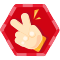## 总结一下C语言中宏的一些特殊用法和几个容易踩的坑。由于本文主要参考GCC文档，某些细节（如宏参数中的空格是否处理之类）在别的编译器可能有细微差别，请参考相应文档。

### 宏基础

#### 1. 标示符别名

`#define BUFFER_SIZE 1024`

```#define NUMBERS 1, \
2, \
3```

#### 2. 宏函数

`#define min(X, Y)  ((X) < (Y) ? (X) : (Y))`

`y = min(1, 2);`会被扩展成`y = ((1) < (2) ? (1) : (2));`

### 宏特殊用法

#### 1. 字符串化(Stringification)

```#define WARN_IF(EXP) \
do { if (EXP) \
fprintf (stderr, "Warning: " #EXP "\n"); } \
while (0)```

`WARN_IF (x == 0);`会被扩展成：

```do { if (x == 0)
fprintf (stderr, "Warning: " "x == 0" "\n"); }
while (0);```

#### 2. 连接(Concatenation)

```#define COMMAND(NAME)  { #NAME, NAME ## _command }

struct command
{
char *name;
void (*function) (void);
};```

```struct command commands[] =
{
COMMAND (quit),
COMMAND (help),
...
};```

```struct command commands[] =
{
{ "quit", quit_command },
{ "help", help_command },
...
};```

### 几个坑

#### 2. 算符优先级问题

`#define MULTIPLY(x, y) x * y`

`MULTIPLY(1, 2)`没问题，会正常展开成`1 * 2`。有问题的是这种表达式`MULTIPLY(1+2, 3)`，展开后成了`1+2 * 3`，显然优先级错了。

`#define MULTIPLY(x, y) (x) * (y)`

`MULTIPLY(1+2, 3)`就会被展开成`(1+2) * (3)`，优先级正常了。

#### 3. 分号吞噬问题

```#define SKIP_SPACES(p, limit)  \
{ char *lim = (limit);         \
while (p < lim) {            \
if (*p++ != ' ') {         \
p--; break; }}}```

```if (*p != 0)
SKIP_SPACES (p, lim);
else ...```

```#define SKIP_SPACES(p, limit)     \
do { char *lim = (limit);         \
while (p < lim) {            \
if (*p++ != ' ') {         \
p--; break; }}}          \
while (0)```

```if (*p != 0)
do ... while(0);
else ...```

#### 4. 宏参数重复调用

`#define min(X, Y)  ((X) < (Y) ? (X) : (Y))`

#### 5. 对自身的递归引用

`#define foo (4 + foo)`

```#define x (4 + y)
#define y (2 * x)```

`x`展开成`(4 + y) -> (4 + (2 * x))``y`展开成`(2 * x) -> (2 * (4 + y))`

#### 6. 宏参数预处理

```#define AFTERX(x) X_ ## x
#define XAFTERX(x) AFTERX(x)
#define TABLESIZE 1024
#define BUFSIZE TABLESIZE```
• `AFTERX(BUFSIZE)`会被展开成`X_BUFSIZE`。因为宏体中含有`##`，宏参数直接代入宏体。
• `XAFTERX(BUFSIZE)`会被展开成`X_1024`。因为`XAFTERX(x)`的宏体是`AFTERX(x)`，并没有`#``##`，所以`BUFSIZE`在代入前会被完全展开成`1024`，然后才代入宏体，变成`X_1024`

-EOF-### shzworkC语言叶子编程
2018/07/27
0
0

2014/10/28
1K
15

Jung_zhang
2015/05/27
0
0
【转】编译器 cc、gcc、g++、CC 的区别

【转】编译器 cc、gcc、g++、CC 的区别 cstriker1407的笔记本2017-12-123 阅读 C编程C++linux 本文转自【 https://www.cnblogs.com/52php/p/5681725.html 】 gcc 是GNU Compiler Collection......

cstriker1407的笔记本
2017/12/12
0
0
Linux下__attribute__((aligned(n)))的使用

fengbingchun
2018/07/31
0
0

Java8新特性，语法学习

package com.example.demo.java8;import com.example.demo.domin.User;import java.util.ArrayList;import java.util.Arrays;import java.util.List;import java.util.stream.Co......

Gx_ww
6分钟前
4
0
type（）和isinstance（）之间有什么区别？

javail
6分钟前
4
0

sunwuhan
9分钟前
2
0
RPA是什么？RPA发展极简史

UiBot
10分钟前
3
0

Hyperledger Fabric和其他许多区块链的关键区别之一，就在于Fabric区块链的交易执行过程：Fabric交易需要首先通过节点的背书，然后再进行交易排序，最后才利用有序交易进行账本的更新。本文将...

12分钟前
3
0